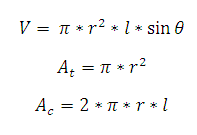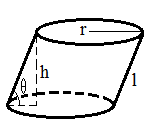# Circular Cylinder Calculator

This CalcTown calculator calculates the volume, top surface area and curved surface area of circular cylinder.

#### Result

m3
m2
m2Where,

π = 3.14 (constant)

r = radius of the cylinder

l = slant height of the cylinder

θ = slant angle

V = volume of the cylinder

At = top surface area of the cylinder

Ac = curved / lateral surface area of the cylinder